# R.C.C T-Beam

Contents

## RCC T-beam:

The beam consists of a flange and a rib in the form of a T, generally made of RC concrete or metal is known as T-beam. The top part of the Slab which acts along the beam to resist the compressive stress is called flange. The part which lies below the slab and resists the shear stress is called rib.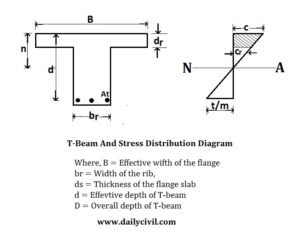## Dimension Of T-beam:

1. The effective width of the flange is adopted as the minimum of c/c distance of the nearby ribs or beams.

2. The overall thickness of the slab crossing over the beam is taken as flange thickness.

3. The breadth of the rib is taken on down earth ground. It should be adequate to hold the steel zone in it, effectively. It might be taken as between 1/3 to 2/3 of the general depth of the beam.

4. The depth of the T-beam is taken between 1/10 tp 1/20 of the span, contingent on the loads acting on it. It can be likewise accepted from the economy point by the given formula: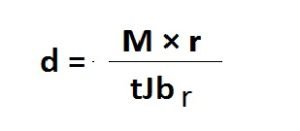Where,

r = Proportion of the cost of steel to the cost of concrete.

M = Maximum bending moment.

## N.A Of T-beam:

The actual NA is calculated by the given formula.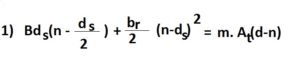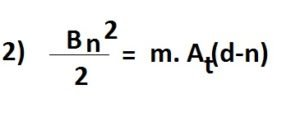Formula 1 is used when the NA is resting under the bottom area of the slab, i.e NA is in the rib and formula 2 is used when NA is resting in the flange of the slab.

As the compressive area of the rib is extremely small, it is always neglected.

## Moment Of Resistance Of T-beam:

Mr = Total compression * Lever arm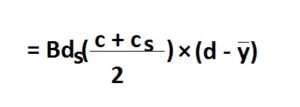Where,

c = compressive stress in the concrete acting bellow the flange.

y = Distance of center of gravity of the compressive force acting under the outermost fiber.The critical NA is calculated by the same equation used for singly reinforced beam.

To determine NA of the T-beam, first, we need to know the area of the steel. It can be evaluated by taking the value of j as 0.9 or on the other hand accepting the center of gravity of the compressive force is lying at mid-depth of the flange slab.